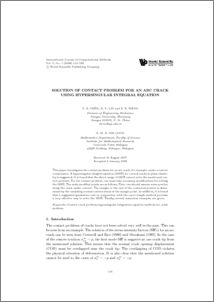# Solution of Contact Problem for an Arc Crack using Hypersingular intergral Equation

## Citation

Chen, Y. Z. and Lin, X. Y. and Wang, Z. X. and Nik Long, Nik Mohd Asri (2008) Solution of Contact Problem for an Arc Crack using Hypersingular intergral Equation. International Journal of Computational Methods, 5 (1). pp. 119-133. ISSN 0219-8762

## Abstract

This paper investigates the contact problem for an arc crack, for example, under a remote compression. A hypersingular integral equation (HSIE) for curved cracks in plane elasticity is suggested. It is found that the direct usage of HSIE cannot solve the mentioned contact problem. For the contact problem, one must take necessary modifications for solving the HSIE. The main modified points are as follows. First, one should assume some portion along the crack under contact. The margin or the end of the contacted portion is determined by the vanishing normal contact stress at the margin point. In addition, it is found that a suggested quadrature rule in conjunction with the curve length method provides a very effective way to solve the HSIE. Finally, several numerical examples are given.Preview
PDF
138.pdfView Item